# What is a pirateplot()?

A pirateplot, is the RDI (Raw data, Descriptive statistics, and Inferential statistics) plotting choice of R pirates who are displaying the relationship between 1 to 3 categorical independent variables, and one continuous dependent variable.

A pirateplot has 4 main elements

1. points, symbols representing the raw data (jittered horizontally)
2. bar, a vertical bar showing central tendencies
3. bean, a smoothed density (inspired by Kampstra and others (2008)) representing a smoothed density
4. inf, a rectangle representing an inference interval (e.g.; Bayesian Highest Density Interval or frequentist confidence interval)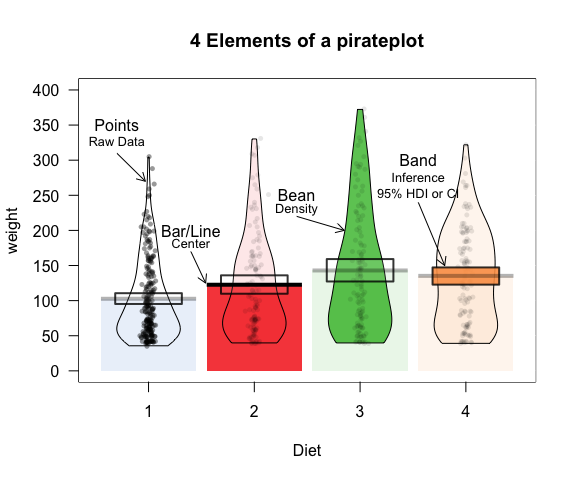# Main arguments

Here are the main arguments to pirateplot()

Main Pirateplot Arguments
Argument Description Examples
formula A formula height ~ sex + eyepatch, weight ~ Time
data A dataframe pirates, ChickWeight
main Plot title ‘Pirate heights’, ’Chicken Weights
pal A color palette ‘xmen’, ‘black’
theme A plotting theme 0, 1, 2
inf Type of inference ‘ci’, ‘hdi’, ‘iqr’

# Themes

pirateplot() currently supports three themes which change the default look of the plot. To specify a theme, use the theme argument:

## Theme 1

theme = 1 is the default

# Theme 1 (the default)
pirateplot(formula = weight ~ Time,
data = ChickWeight,
theme = 1,
main = "theme = 1")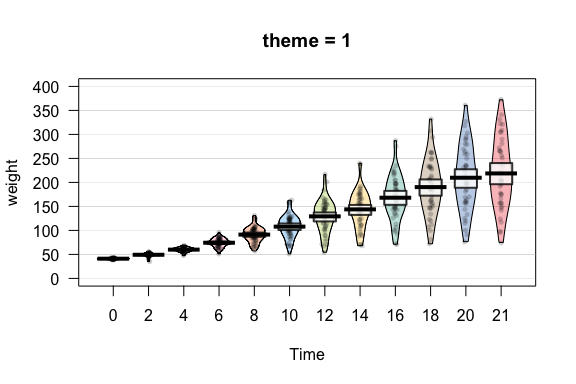## Theme 2

Here is theme = 2

# Theme 2
pirateplot(formula = weight ~ Time,
data = ChickWeight,
theme = 2,
main = "theme = 2")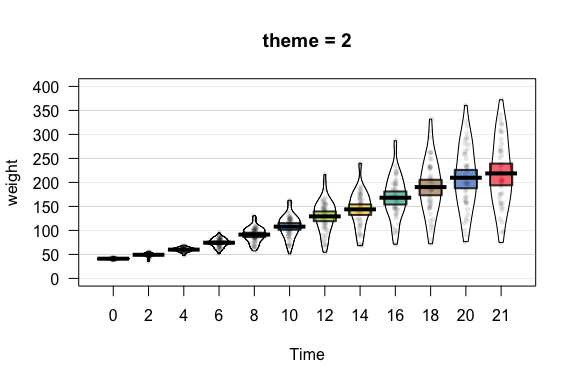## Theme 3

And now…theme = 3!

# Theme 3
pirateplot(formula = weight ~ Time,
data = ChickWeight,
theme = 3,
main = "theme = 3")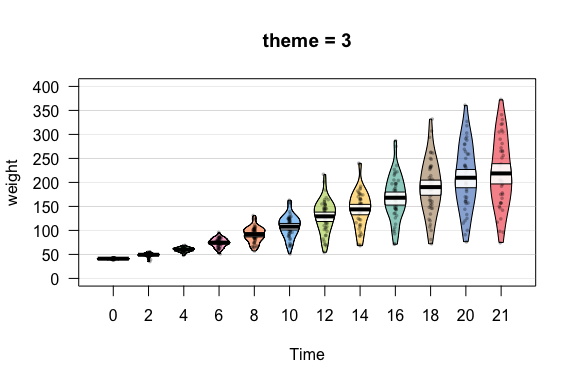## Theme 4

theme = 4 tries to maintain a classic barplot look (but with added raw data).

# Theme 4
pirateplot(formula = weight ~ Time,
data = ChickWeight,
theme = 4,
main = "theme = 4")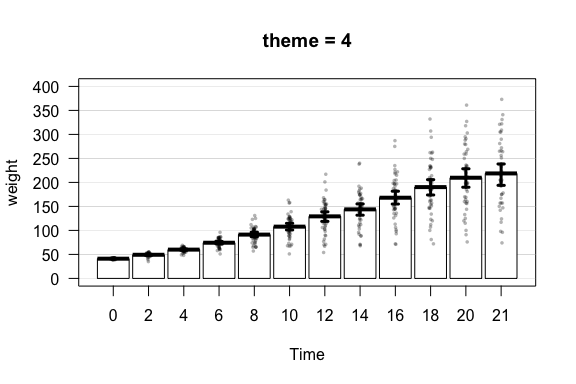## Theme 0

theme = 0 allows you to start a pirateplot from scratch – that is, it turns of all elements. You can then selectively turn elements on with individual arguments (e.g.; bean.f.o, point.o)

# Default theme
pirateplot(formula = weight ~ Time,
data = ChickWeight,
theme = 0,
main = "theme = 0\nStart from scratch")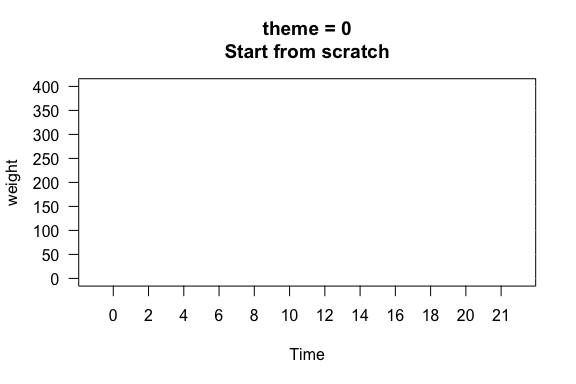# Color palettes

You can specify a general color palette using the pal argument. You can do this in two ways.

The first way is to specify the name of a color palette in the piratepal() function. Here they are:

piratepal("all")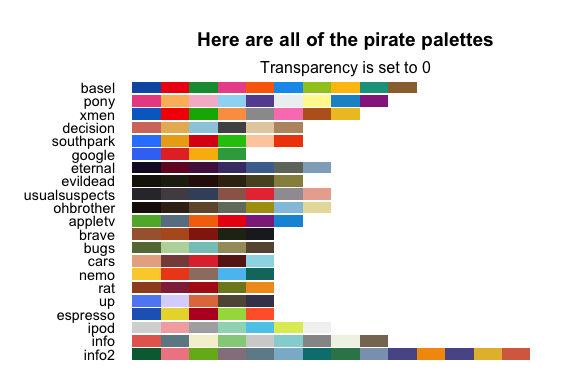For example, here is a pirateplot using the "pony" palette

pirateplot(formula = weight ~ Time,
data = ChickWeight,
pal = "pony",
theme = 1,
main = "pony color palette")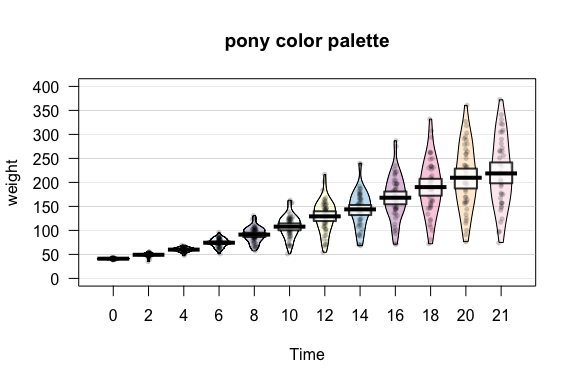The second method is to simply enter a vector of one or more colors. Here, I’ll create a black and white pirateplot from theme 2 by specifying pal = 'black'

pirateplot(formula = weight ~ Time,
data = ChickWeight,
theme = 2,
pal = "black",
main = "pal = 'black")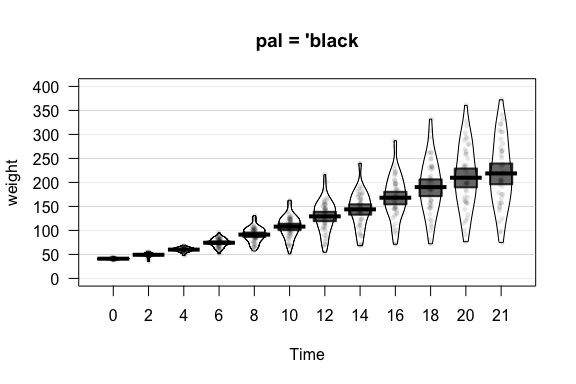# Customising elements

Regardless of the theme you use, you can always customize the color and opacity of graphical elements. To do this, specify one of the following arguments. Note: Arguments with .f. correspond to the filling of an element, while .b. correspond to the border of an element:

Customising plotting elements
element color opacity
points point.col, point.bg point.o
beans bean.f.col, bean.b.col bean.f.o, bean.b.o
bar bar.f.col, bar.b.col bar.f.o, bar.b.o
inf inf.f.col, inf.b.col inf.f.o, inf.b.o
avg.line avg.line.col avg.line.o

For example, I could create the following pirateplots using theme = 0 and specifying elements explicitly:

pirateplot(formula = weight ~ Time,
data = ChickWeight,
theme = 0,
main = "Fully customized pirateplot",
pal = "southpark", # southpark color palette
bean.f.o = .6, # Bean fill
point.o = .3, # Points
inf.f.o = .7, # Inference fill
inf.b.o = .8, # Inference border
avg.line.o = 1, # Average line
bar.f.o = .5, # Bar
inf.f.col = "white", # Inf fill col
inf.b.col = "black", # Inf border col
avg.line.col = "black", # avg line col
bar.f.col = gray(.8), # bar filling color
point.pch = 21,
point.bg = "white",
point.col = "black",
point.cex = .7)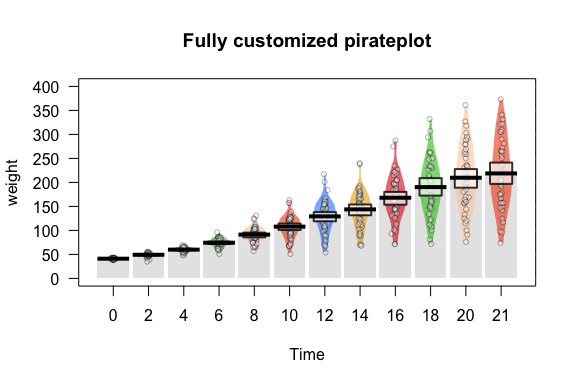If you don’t want to start from scratch, you can also start with a theme, and then make selective adjustments:

pirateplot(formula = weight ~ Time,
data = ChickWeight,
main = "Adjusting an existing theme",
inf.f.o = 0, # Turn off inf fill
inf.b.o = 0, # Turn off inf border
point.o = .2,   # Turn up points
bar.f.o = .5, # Turn up bars
bean.f.o = .4, # Light bean filling
bean.b.o = .2, # Light bean border
avg.line.o = 0, # Turn off average line
point.col = "black" # Black points
)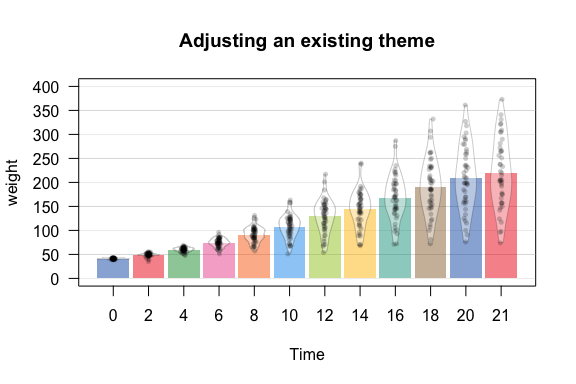Just to drive the point home, as a barplot is a special case of a pirateplot, you can even reduce a pirateplot into a horrible barplot:

pirateplot(formula = weight ~ Time,
data = ChickWeight,
main = "Reducing a pirateplot to a barplot",
theme = 0, # Start from scratch
bar.f.o = .7) # Just turn on the bars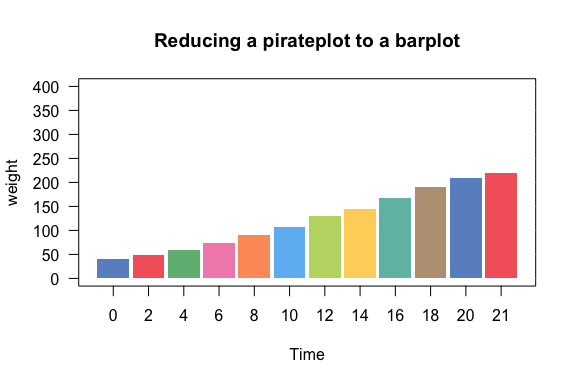There are several more arguments that you can use to customize your plot:

element arguments examples
Background color back.col back.col = ‘gray(.9, .9)’
Gridlines gl.col, gl.lwd, gl.lty gl.col = ‘gray’, gl.lwd = c(.75, 0), gl.lty = 1
Quantiles quant, quant.lwd, quant.col quant = c(.1, .9), quant.lwd = 1, quant.col = ‘black’
Average line avg.line.fun avg.line.fun = median
Inference Calculation inf.method inf.method = ‘hdi’, inf.method = ‘ci’
Inference Display inf.disp inf.disp = ‘line’, inf.disp = ‘bean’, inf.disp = ‘rect’

Here’s an example using a background color, and quantile lines.

pirateplot(formula = weight ~ Time,
data = ChickWeight,
main = "Adding quantile lines and background colors",
theme = 2,
back.col = gray(.98), # Add light gray background
gl.col = "gray", # Gray gridlines
gl.lwd = c(.75, 0),
inf.f.o = .6, # Turn up inf filling
inf.disp = "bean", # Wrap inference around bean
bean.b.o = .4, # Turn down bean borders
quant = c(.1, .9), # 10th and 90th quantiles
quant.col = "black" # Black quantile lines
)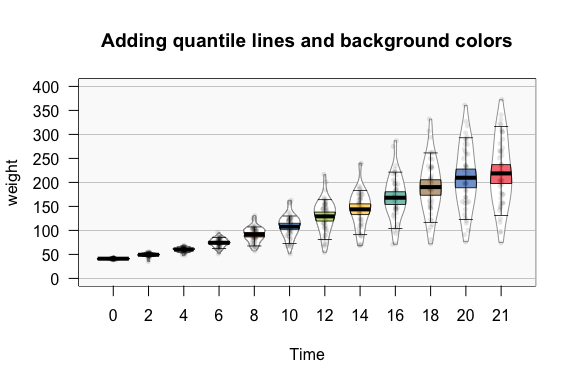# Multiple IVs

You can use up to 3 categorical IVs in your plot. Here are some examples:

pirateplot(formula = height ~ sex + eyepatch + headband,
data = pirates,
theme = 2,
inf.disp = "bean")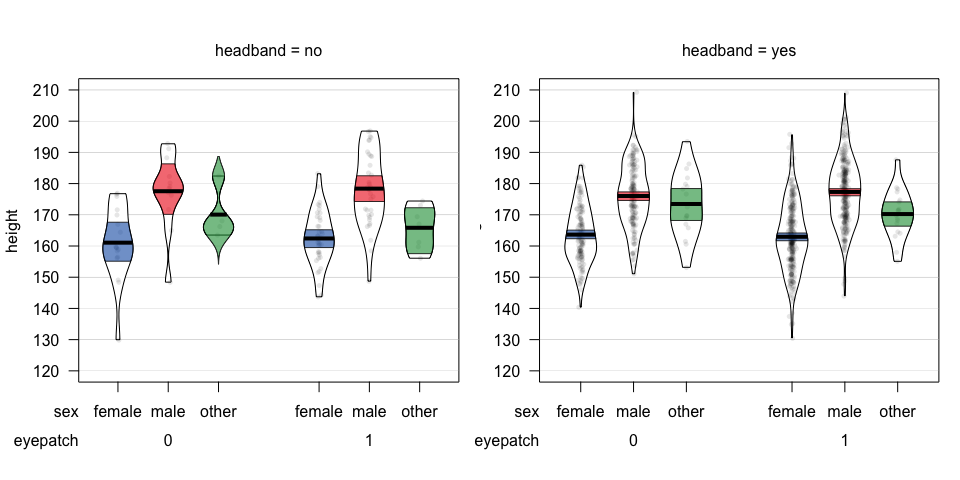Here’s a pirateplot with showing the relationship between movie running times based on movie genre and whether the movie is a sequel or not.

pirateplot(formula = time ~ sequel + genre + rating,
data = subset(movies,
genre %in% c("Action", "Adventure", "Comedy", "Horror") &
rating %in% c("G", "PG", "PG-13", "R") &
time > 0),
theme = 3,
cex.lab = .8,
inf.disp = "rect",
pal = "up")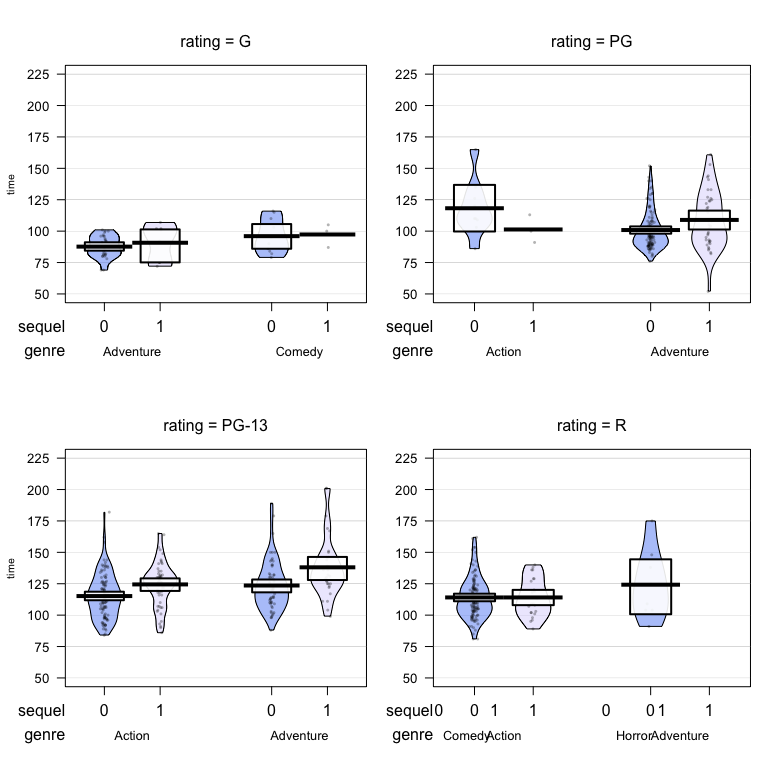# Output

If you include the plot = FALSE argument to a pirateplot, the function will return some values associated with the plot.

times.pp <- pirateplot(formula = time ~ sequel + genre,
data = subset(movies,
genre %in% c("Action", "Adventure", "Comedy", "Horror") &
rating %in% c("G", "PG", "PG-13", "R") &
time > 0),
plot = FALSE)

Here’s the result. The most interesting element is $summary which shows summary statistics for each bean: times.pp ##$summary
##   sequel     genre bean.num   n       avg    inf.lb   inf.ub
## 1      0    Action        1 233 114.73391 112.54208 116.9171
## 2      1    Action        2  80 120.47500 116.47585 124.5282
## 3      0 Adventure        3 206 106.36408 103.33926 109.1894
## 4      1 Adventure        4  78 118.64103 111.37081 124.0271
## 5      0    Comedy        5 400 102.01500 100.92166 103.1729
## 6      1    Comedy        6  51 101.21569  98.31366 103.8705
## 7      0    Horror        7  79 102.13924  98.43406 105.3444
## 8      1    Horror        8  23  97.65217  92.99968 102.9126
##
## $avg.line.fun ##  "mean" ## ##$inf.method
##  "hdi"
##
## \$inf.p
##  0.95

# Contribute!

I am very happy to receive new contributions and suggestions to improve the pirateplot. If you come up a new theme (i.e.; customization) that you like, or have a favorite color palette that you’d like to have implemented, please contact me (yarrr.book@gmail.com) or post an issue at www.github.com/ndphillips/yarrr/issues and I might include it in a future update.